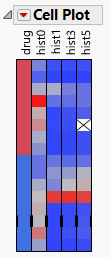Essential Graphing > Cell Plots > The Cell Plot
Publication date: 05/24/2021

# The Cell Plot

The cell plot appears with a one-to-one correspondence of a colored cell representing each data table entry. Colors are assigned to each cell based on the range and type of values found in the column.

Note: Any rows that are excluded in the data table are also hidden in the cell plot.

Figure 8.4 The Cell PlotNote: To produce the plot shown in Figure 8.4, follow the instructions in Example of a Cell Plot.

Note the following information about cell plots:

Nominal variables use a distinct color for each level. You can customize nominal and ordinal colors using the Value Colors property of data columns, available through the Column Info command.

Continuous variables are assigned a gradient of colors to show the smooth range of values in the variable.

Ordinal variables are scaled like continuous variables in order.

When some outliers are present, the scale uses all but the extreme categories for the 90% middle of the distribution, so that the outliers do not overly influence the scale.

Related Information